So, how do we check to see if two functions are inverses of each other?

Well, we learned before that we can look at the graphs.  Remember, if the two graphs are symmetric with respect to the line y = x (mirror images over y = x ), then they are inverse functions.

But, we need a way to check without the graphs, because we won't always know what the graphs look like!

So, just crunching some Algebra, here's one way to look at it:

 If you're got two functions, f(x) and g(x), and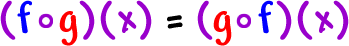then f(x) and g(x) are inverse functions.

Let's try this on an easy one that we know will work: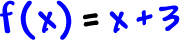and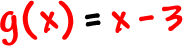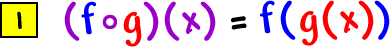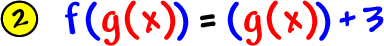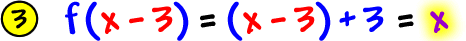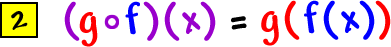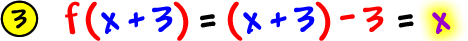So,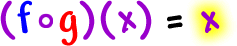and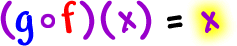Yep, they are inverses, just like we thought!